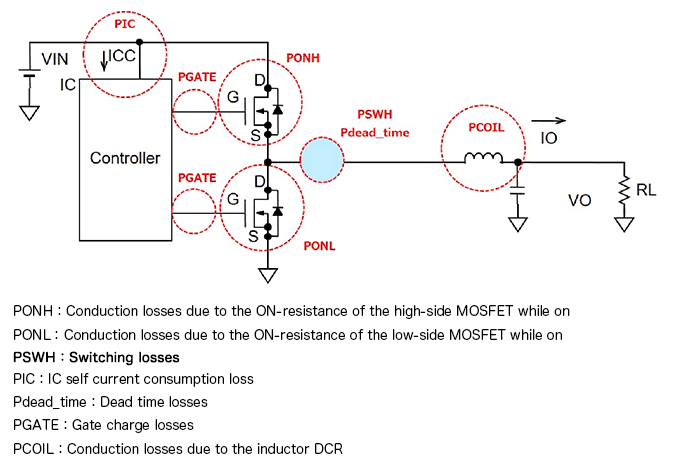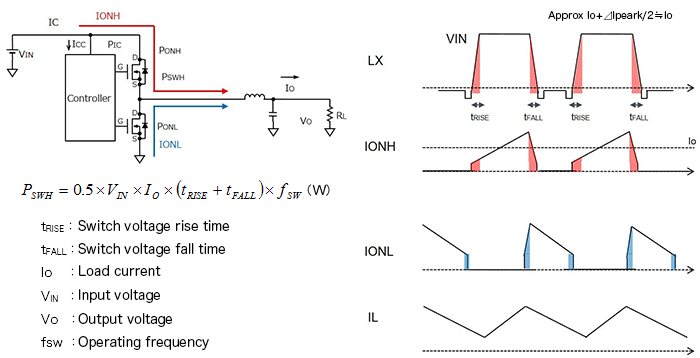# Switching Losses in Synchronous Rectifying Step-Down Converters

2018.09.06

・The switching loss in a synchronous rectifying step-down converter is calculated using the switching transition time, the power during this transition time, and the switching frequency.

In the preceding article, we examined conduction losses in output-stage MOSFETs that are power switches in synchronous rectifying step-down converters. This time, we consider switching losses occurring at switch nodes.## Switching Losses

Switching losses, as the name suggests, are losses that accompany switching operation. The symbol PSWH is used.

A synchronous switch (high-side or low-side) of a synchronous rectifying step-down converter can be schematically thought of as switching (turning on/off) the voltages VIN and GND. The value obtained simply by multiplying the power in this transition time by the switching frequency is the switching loss. Refer to LX in the waveform diagrams below. The loss in time intervals in which the device is turned completely on or off is the conduction loss, explained in the previous article.

PSWH can be calculated using the following equation.The power in the triangular area the base of which is the rise time or fall time and the height of which is VIN, is the loss. The faster the rise or fall time, the smaller is the loss during the transition time, but the switching frequency factor is present, and so it must be remembered that in order to reduce the switching loss, both the switching transition time and the switching frequency must be considered.

Next time, we will discuss dead-time losses.